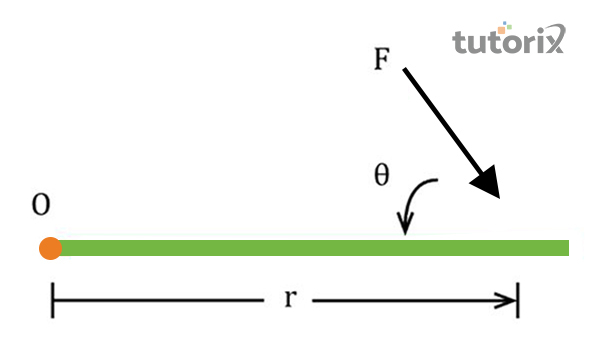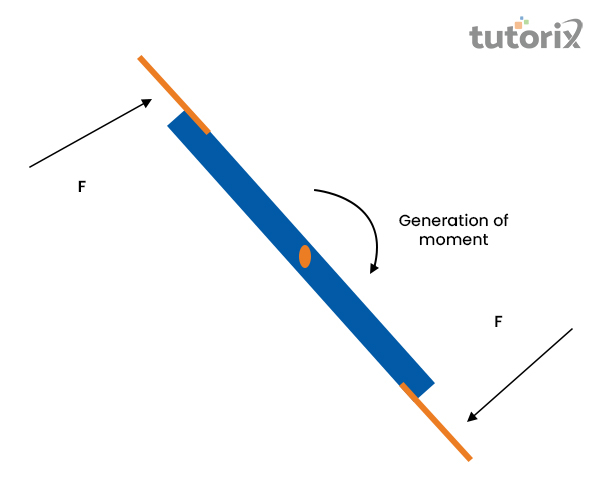# Difference Between torque and Moment

## Introduction

The terms torque and moment have different meaning and both of them differs from each other. It is seen that torque is a form and it is multiplies by distance. Moment refers to the force multiplies by any distance from the body. It is seen that moment turns due to the force of rotation. The main difference between these two factors is that torque is the measurement of turning force and moment is a measurement of perpendicular distance.

### Definition of TorqueFigure 1: Torque

Torque is nothing but the measurement of the force of an object. This force in this field helps to rotate the object. It is seen that the rotation of the object happens within its axis. It can be marked as the pivot point (O). Here force is named as (F) and r is the name of moment arm (Physics, 2022).

Moment arm mainly refers to the difference between the pivot point to the point where the force acts. Torque here can be expressed through a formula and it is $\mathrm{\Gamma = r \times F = rFsin(\Theta)}$. The formula also defines the matter that torque is the cross product of distance vector and force vector. The most important factor here is that torque causes an angular acceleration. Torque on the other hand can be called the rotational equivalent of linear force.

## Definition of MomentFigure 2: Moment

Moment in physics is mainly referred to as the moment of a force. It is the measurement of its tendency and it created the body to move around the specific point or the axis. Here moment of force can be described as the effect of rotation f a force. It is related to the force’s size and lies at a perpendicular distance. Mainly three types of the moment can see in physics (Merriam-webster, 2022).

They are Polar moment of inertia, Area moment of inertia, and Mass moment of inertia. An example of a moment can be the opening of a door. It is seen that when the door is opened by applying force on the handle, then it is an example of a moment.

### Differences between Torque and Moment

Sl no Torque Moment
1. Torque refers to the twisting and measure of turning an object. Moment is the perpendicular length between the force of action and the point of turn (Askanydifference, 2022).
2. It mainly infers the force that rotates the object. Moment is the force that helps to move the object.
3. It is a moment-relevant force. It is the static force
4. Torque is mainly dynamic in nature. Moment is mainly static in nature.
5. It is seen that torque can help to create de-acceleration or angular acceleration. Moment in this field is not able to do these things.
6. It is applied when there is rotation within the object. It is a non-rotational movement.

Table 1: Differences between Torque and Moment

### Static and Dynamic Torque

Static torque can be defined as a torque that is incapable of producing the ‘angular acceleration in real-time. When someone is pushed on a closed-door and static torque is applied to them, the door does not rotate in its hives despite the amount of force applied to it. For example, when someone is riding a bicycle at a certain and constant speed, static torque is being applied here generally as they are not accelerating in real-time (Memon et al. 2020). In simple words, if a torque can produce a reactive force it can be considered as a static torque.

Dynamic torque on the other hand, is a type of torque that is capable of producing an angular acceleration while functioning in real-time. When someone driving a racing car starts accelerating, it consequently starts carrying a dynamic torque depending on the production of acceleration at that moment. In that situation, the wheels of the given car can be seen producing accelerations along with the track it is operating on.

### Elements and measurements of the moment

Moment generally consists of two basic elements, force and distance applied to a specific object in real-time. Therefore, moment can be defined as the product of the force (F) and the moment arm (d) that has a perpendicular distance between the centre of moments and the line of action (Abel et al. 2020). The measurement process of moment can be done with the SI-unit of Newton-metre (Nm) which specifically gives direction to a force in the parallel axis of rotation. The measurement also follows the formula of $\mathrm{ \Gamma = F \times d}$ to outline the moment in real-time objects.

## Conclusion

Torque can be defined as the way of measuring the force of an object that is moving in real-time. Moment on the other hand, is the way of measuring the tendency of an object that is moving around a particular axis in real-time. Despite the dependency on each other, there are specific differences like being a moment-relevant force and a static force that can be seen in real-time. Torque is generally dynamic in nature whereas moment is naturally static.

### FAQs

Q1. What is the common relationship between moment and torque?

Torque can be defined as the measurement of turning force within a body and moment appears as the perpendicular distance from one specific point to another when the body faces the line of action So, despite the differences, torque and moment is related to each other in terms of the rotation to the line of action of a force.

Q2. Does torque have a particular direction?

Torque is capable of giving a line of action of force in real-time which eventually allows us to measure the perpendicular distance to the line of action. It eventually allows torque to give a perpendicular direction and a location to the line of action in real-time.

Q3. What happens when the torque becomes zero?

Torque becomes rotational equilibrium when the net torque becomes totally zero. In addition, it becomes incapable of acquiring the angular acceleration when the net torque becomes zero in real-time.